## 3.3.1 POISSON's Equation

To find a discrete approximation of MAXWELL's fourth equation, eqn. (2.4) is integrated over a control volume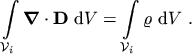(3.18)

Applying the theorem of GAUSS to the left hand side turns eqn. (3.18) into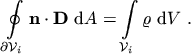(3.19)

The integrals are approximated as follows: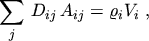(3.20)

where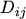is the projection of the flux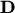onto the grid edge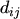, evaluated at the midpoint of the edge,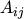is the boundary line which belongs to both subdomainsand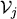, and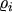is the space charge density at the grid point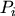(Fig. 3.4).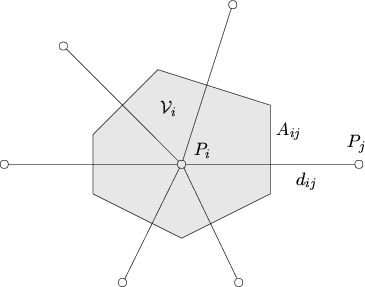The remaining task is to find an approximation for the projection of the dielectric flux density. This is done by the finite difference approximation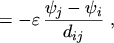(3.21)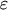(3.22)

With eqn. (3.20) and eqn. (3.21), the discretization of POISSON's equation can be concluded.

M. Gritsch: Numerical Modeling of Silicon-on-Insulator MOSFETs PDF